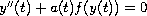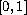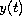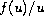Electron. J. Diff. Equ., Vol. 2009(2009), No. 165, pp. 1-8.

### An optimal existence theorem for positive solutions of a four-point boundary value problem Man Kam Kwong, James S. W. Wong

Abstract:
We are interested in the existence of positive solutions to a four-point boundary value problem of the differential equationon. The value ofat 0 and 1 are each a multiple ofat an interior point. Many known existence criteria are based on the limiting values ofasapproachesand infinity.
In this article we obtain an optimal criterion (thereby improving all existing results of kind mentioned above) by comparing these limiting values to the smallest eigenvalue of the corresponding four-point problem of the associated linear equation. In the simpler case of three-point boundary value problems, the same result has been established in an earlier paper by the first author using the shooting method.
The method of proof is based upon a variant of Krasnoselskii's fixed point theorem on cones, the classical Krein-Rutman theorem, and the Gelfand formula relating the spectral radius of a linear operator to its norm.

Submitted February 12, 2009. Published December 22, 2009.
Math Subject Classifications: 34B10, 34B15, 34B18.
Key Words: Four-point boundary value problem; second-order ODE; Krasnoselskii fixed point theorem; mappings on cones.

Show me the PDF file (205 KB), TEX file, and other files for this article.Man Kam Kwong Department of Applied Mathematics Hong Kong Polytechnic University, Hong Kong, SAR, China email: mankwong@polyu.edu.hk James S. W. Wong Institute of Mathematical Research Department of Mathematics, University of Hong Kong Hong Kong, SAR, China email: jsww@chinneyhonkwok.com